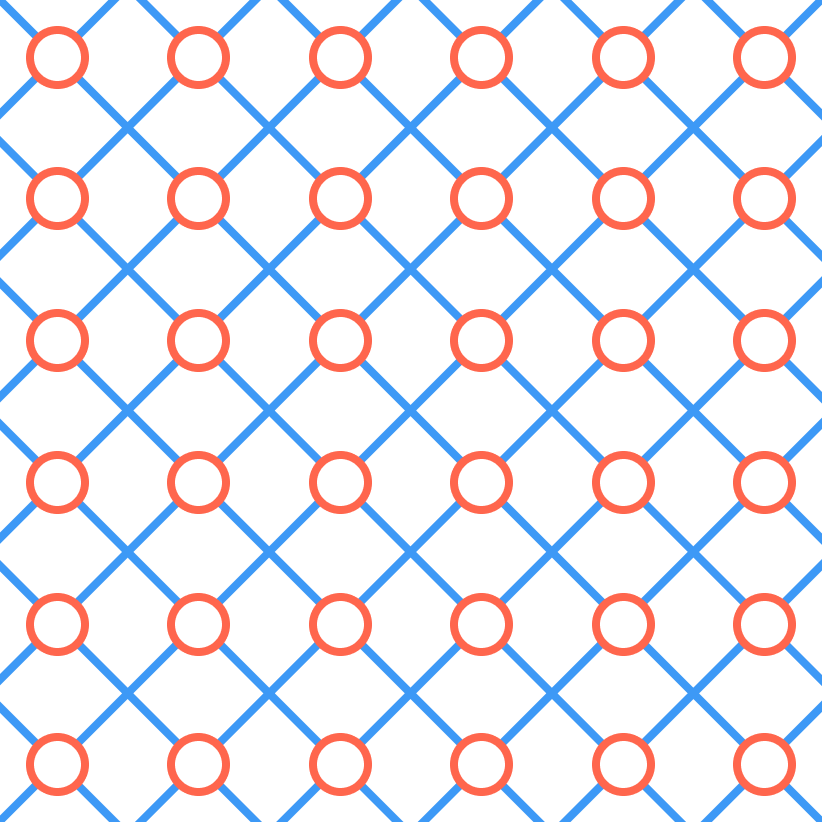# $6\times 6$

Write one number in each red circle so that the sum of the numbers along each blue, diagonal line is always the same number $x.$

Find the maximum value of $x$.×